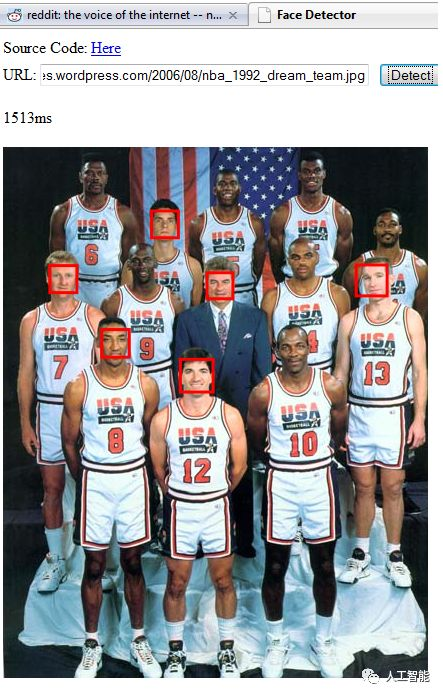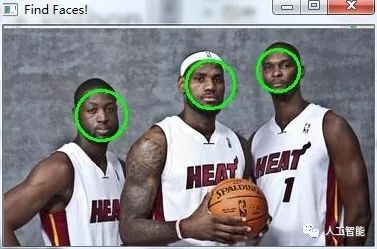• 服务热线：0755-84872125
• wangyp@shangeai.com

# 基于OpenCV和 Python的人脸识别实现方案## 必备知识

Haar-like

Haar-like百科释义。通俗的来讲,就是作为人脸特征即可。

Haar特征值反映了图像的灰度变化情况。例如:脸部的一些特征能由矩形特征简单的描述,如:眼睛要比脸颊颜色要深,鼻梁两侧比鼻梁颜色要深,嘴巴比周围颜色要深等。

opencv api

import cv2gray = cv2.cvtColor(image,cv2.COLOR_BGR2GRAY)

opencv 的强大之处的一个体现就是其可以对图片进行任意编辑,处理。 下面的这个函数最后一个参数指定的就是画笔的大小。

import cv2cv2.rectangle(image,(x,y),(x+w,y+w),(0,255,0),2)

import cv2cv2.imshow("Image Title",image)

import cv2

# 探测图片中的人脸

faces = face_cascade.detectMultiScale( gray, scaleFactor = 1.15, minNeighbors = 5, minSize = (5,5), flags = cv2.cv.CV_HAAR_SCALE_IMAGE)

import cv2

print "发现{0}个人脸!".format(len(faces))

for(x,y,w,h) in faces: cv2.rectangle(image,(x,y),(x+w,y+w),(0,255,0),2)

## 实例# coding:utf-8

import sys

sys.setdefaultencoding('utf8')

# __author__ = '郭 璞'

# __date__ = '2016/9/5'

# __Desc__ = 人脸检测小例子,以圆圈圈出人脸

import cv2

# 待检测的图片路径

imagepath = r'./heat.jpg'

# 获取训练好的人脸的参数数据,这里直接从GitHub上使用默认值

# 读取图片

gray = cv2.cvtColor(image,cv2.COLOR_BGR2GRAY)

# 探测图片中的人脸

gray,

scaleFactor = 1.15,

minNeighbors = 5,

minSize = (5,5),

flags = cv2.cv.CV_HAAR_SCALE_IMAGE

)

print "发现{0}个人脸!".format(len(faces))

for(x,y,w,h) in faces:

# cv2.rectangle(image,(x,y),(x+w,y+w),(0,255,0),2)

cv2.circle(image,((x+x+w)/2,(y+y+h)/2),w/2,(0,255,0),2)

cv2.imshow("Find Faces!",image)

cv2.waitKey(0)D:\Software\Python2\python.exe E:/Code/Python/DataStructor/opencv/Demo.py发现3个人脸!

• 标签: